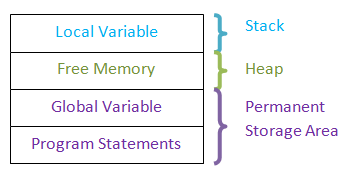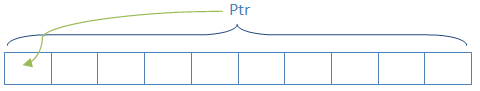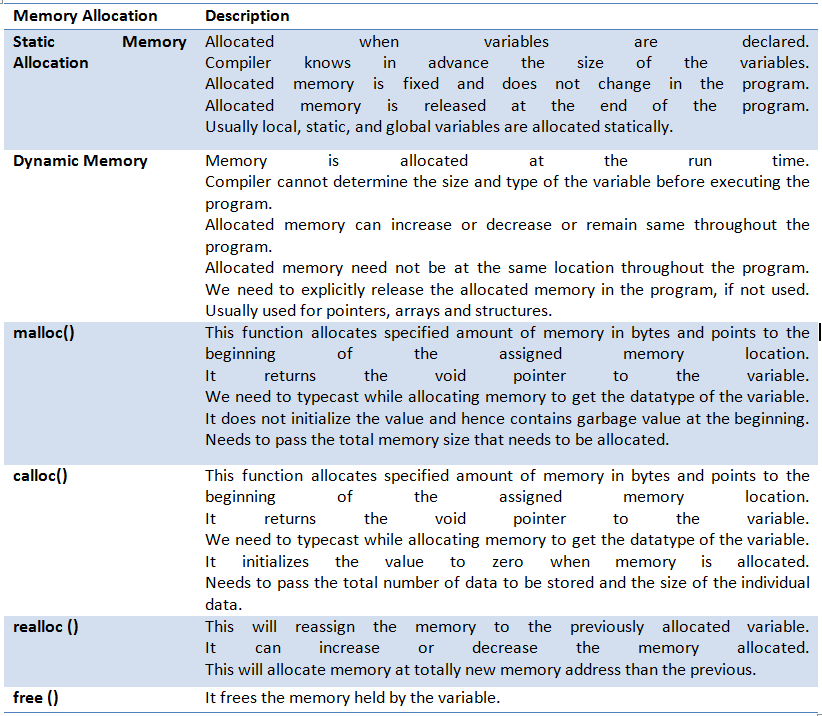# Memory Management in C Programming

## Introduction

Every programming language deals with memory in the system. Each and every variable needs a specified amount of memory, the program itself require memory to store its own program, some temporary memory to store intermediate values etc. Hence it is required to manage the memory with utmost care. Memory locations assigned to one program or variable should not be used by another program or variable. He.nce C provides 2 methods of allocating memory to the variables and programs. They are static and dynamic memory allocations. In static memory allocation, memory is allocated at the time of compilation and will be same throughout the program. There will not be any changes to the amount of memory nor the location in the memory. But in the case of dynamic memory allocation, memory is allocated at the run time and we can increase/decrease the amount of memory allocated or completely release the memory when not in use. We can reallocate the memory when it is required. Hence dynamic memory allocation gives the flexibility to use the memory efficiently.

Before proceeding to memory allocation, let us understand types of variables, types of memory and methods of allocating memory to the various variables and programs. In a program, we will have different types of variables and memory requirement. The global variables are the ones which will be used throughout the program by different functions and blocks. Hence memory area allocated to them needs to exist throughout the program. Hence they get memory allocated at the internal memories of the system, which are known as permanent storage area. Similarly the program and their statements also need to exist throughout when system is on. Hence they also need to occupy permanent storage area.

Local variables are the one which need to exist in the particular block or function where they are declared. If we store them in permanent storage area, it will be waste of memory as we keep the memory allocate which are not in use. Hence we use stack memory to store the local variables and remove them from the stack as the use of local variable is over.

There is a free memory space between this stack memory and permanent storage area called heap memory. This memory is flexible memory area and keeps changing the size. Hence they are suitable for allocating the memory during the execution of the program. That means dynamic memory allocations use these heap memories.## Static Memory Allocation

Suppose we need to add two integer numbers and display the result. Here we know how many variables and which type of variables are involved in calculations. i.e.; we need two integer variables to store two numbers and one integer variable to store result. Thus we need three integer variables. This implies that at compile time itself we know that there are 3 integer variables. Hence it is easy for the compiler to reserve the memory for these variables. Such reserved variables will have same size and memory address till the end of the program. There will not be any change in size, type and memory location for those variables.

This kind of memory allocation for the variables is known as static memory allocation. Here no need to explicitly allocate memory to the variables. When we declare the variables, memory will be automatically assigned to them. These variables can be local or global variables. But we need to know in advance the size and type of the variable. They need not be simple variables; but they can be array or structure too provided we know their size.

```int intX; // needs to be initialized or assigned some value at run time
int intExample = 0; //normal variable
const int intConstant = 10; // constant, read-only variable```

In above all cases the compiler knows in advance that they are also integers and their size. Hence compiler will allocate specific memory locations before executing the program itself. These allocated memories will not be freed until the program execution is over. These allocated memory location and their size is constant throughout the program. Any these kinds of variables cannot store more than predefined size of data in this case.

## Dynamic Memory Allocation

This is in contrast to the static memory allocation. Here program will not know the size and even sometimes type of the variable. It is determined only at the execution time. In such case, we cannot assign any memory at compilation time. It can be assigned only at the run time.

Suppose we need to add any number of numbers that are entered by the user. Here we are not sure about how many numbers are entered by the user. We only know that it he is entering only integers. In this case we cannot pre-assign any memory to the variables. He can enter only 2 numbers or 100s of numbers. If user enters less numbers then the program should be flexible enough to assign to those less number of numbers and as the numbers increase memory allocation space also should increase. But this can be determined only at the run time – depends on the user who enters the value. Thus we need to allocate space at the run time which is done by using dynamic memory allocation methods.

There are different functions to allocate memory to the variables at run time and fluctuate the size of the memory for the variables. The best example of dynamic memory allocation is pointers, structures and arrays. Here we will not be aware of number of variables and types of variables being used. We can assign memory and determine the type of the variable at run time using below functions.

### malloc ()

this is the most common method of allocating memory at run time. This function allocates requested amount of memory to the variables at run time and returns the void pointer to the first memory address. That means it allocates the requested amount of memory in bytes and it does not points/ defines datatype for the variable. It considers the variable as void and moves its pointer to the first byte in the allocated memory. In case it cannot allocate memory, then it returns the NULL pointer. When memory is allocated using malloc, variables not initialized t

The general syntax for allocating memory using malloc is:

(cast_type *) malloc (size_in_bytes);
ptr =    malloc(10); // allocates 10 bytes of memory

Here ptr is a pointer variable and it allocates 10 bytes of memory. Here we have not defined datatype of the variable and ptr is a void pointer now. It will now point to the first byte in the allocated memory.If we need to make this pointer as integer then we need specify the type also while allocating memory. If we do this, then when we assign values, the values will be stored at the interval of those many sizes. That means, if we make ptr as integer and start storing the data, then each data value will be stored at the interval of 4 bytes.

`ptr = (int*)malloc(10); //returns integer pointer to ptr pointing to first byte of allocated memory`

This will make ptr as integer pointer and allocated memory is only 10 bytes. If it is divided for integer value (4 bytes each), then we will be able to store only 2 values. But the size of the integer may vary from system to system. Hence we can allow processor itself to determine the size of the integer and allocate memory to the pointer. In addition, we can specify how many data value of integer size needs to be stored.

`ptr = (int*)malloc(10* sizeof(int)); //allocates memory sufficient for 10 integer values and returns integer pointer to ptr`

The parameter within malloc will have total size of memory to be allocated. But we cannot calculate the total size always. Hence we perform calculation with malloc to determine the total size of the memory to be allocated.

Suppose we need to create a dynamic array of floating numbers to store 100 elements. Then:

`arr = (float*)malloc(10 * sizeof(float));`

Same method can be used to allocate memory for the structures. Suppose we have student structure. Then:

`struct student *std = (struct student *)malloc(sizeof(struct student));`

Here structure pointer *std is allocated memory dynamically. It gets the memory allocated to store one student details and makes the pointer to point to the first member of the student structure. Suppose same *std should hold more than one student – say 50 students. Then we need to allocate memory to hold 50 * sizeof (student).

`struct student *std = (struct student *)malloc(50 * sizeof(struct student));`

### calloc ()

This function is similar to malloc. But this function is usually used to allocate memories to arrays and structures. When calloc () is used to allocate memory, it automatically initializes the variable to zero. Suppose we need to allocate memory for 50 students. In malloc we multiply 50 with the size of student structure to get the total memory size. But in calloc, we pass 50 and size of student as two arguments as shown below. Apart from this, it allocates memory in the same way as malloc.

```(cast_type *) calloc (blocks , size_of_block);
struct student *std = (struct student *)calloc(sizeof(struct student));// single student
struct student *std = (struct student *)malloc(50, sizeof(struct student));// 50 students```

### realloc ()

Suppose we need to increase or decrease the memory size of already allocated variable. In such case we can use realloc function to re-define memory size of the variable.

(cast_type *) realloc (blocks, size_of_block);
It will allocate totally new memory location with new block size.

### free ()

It is always good practice to release the allocated memory once it is no longer required. This is because whenever the memory is allocated dynamically, they will consume lot of memory space to the variables. It will be available to same or different programs only when it is released. However, all the memories held by the program will be released automatically, once the program is complete.

free (variable_name);
free (std);

## SummaryTranslate »